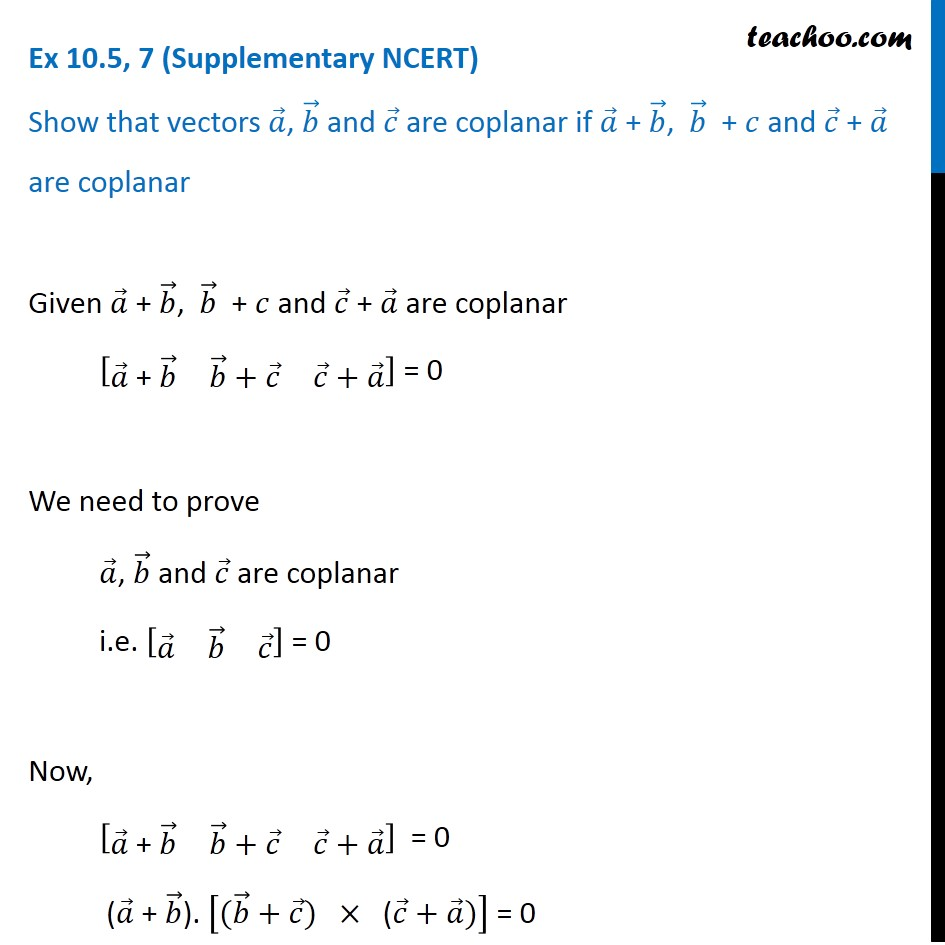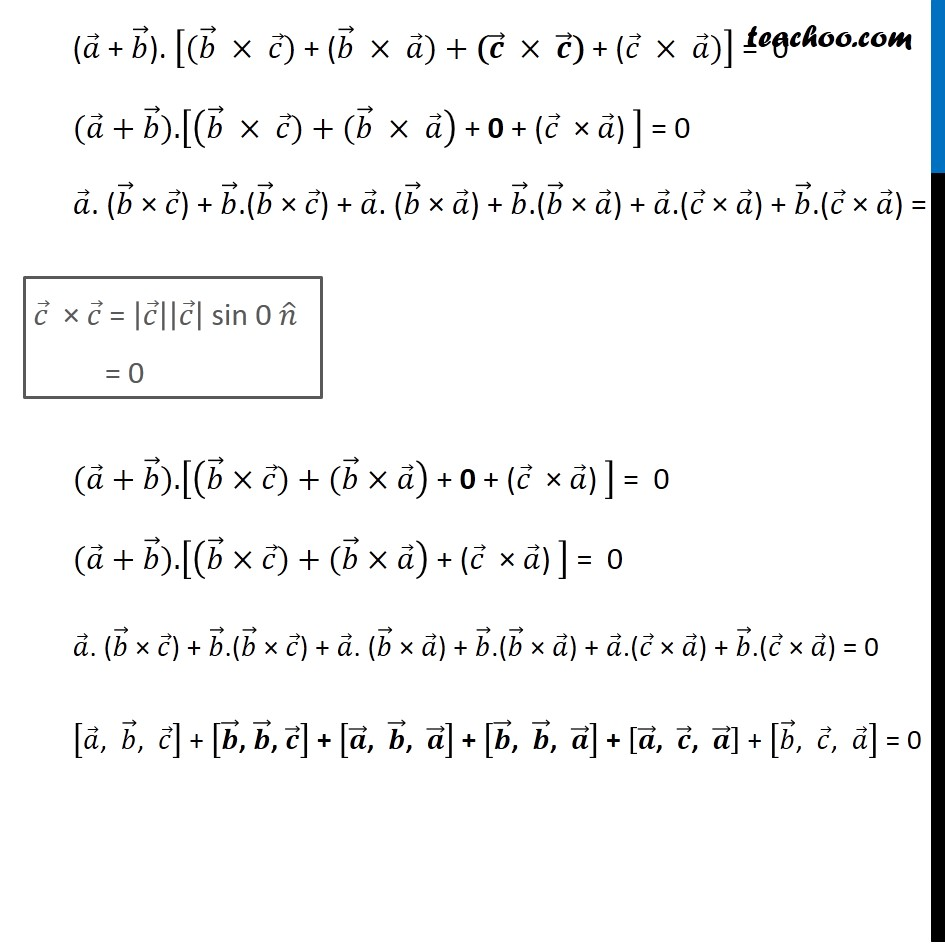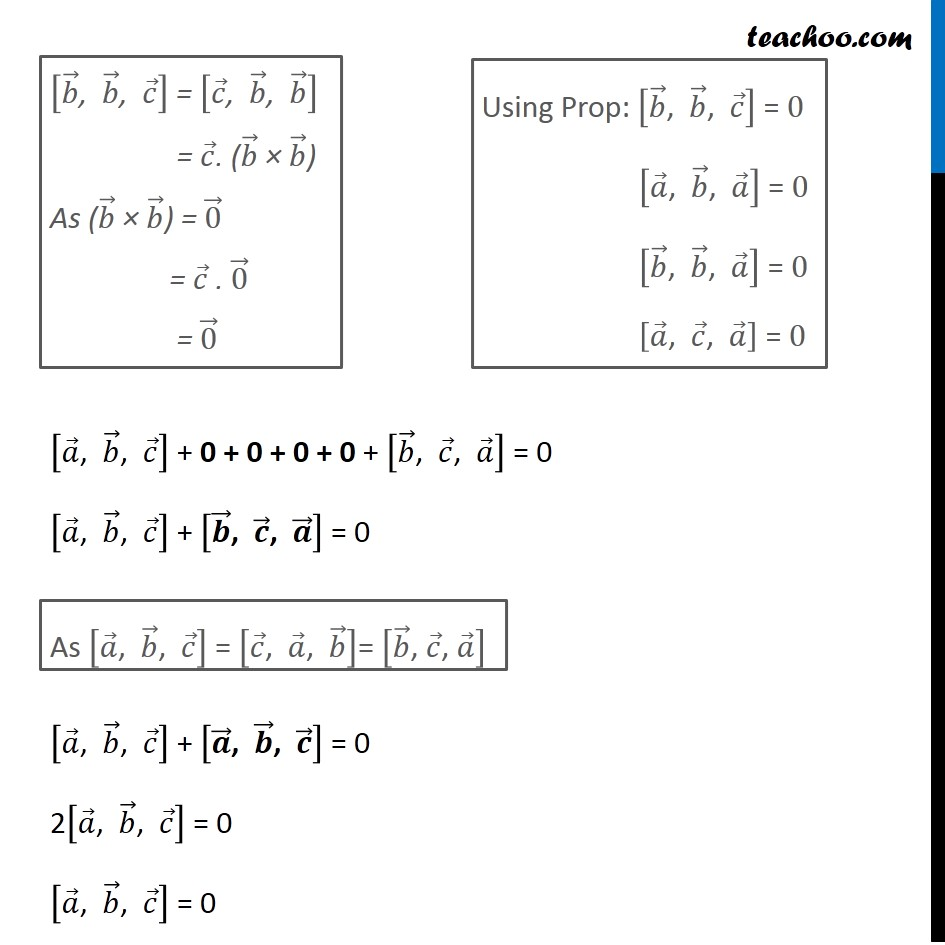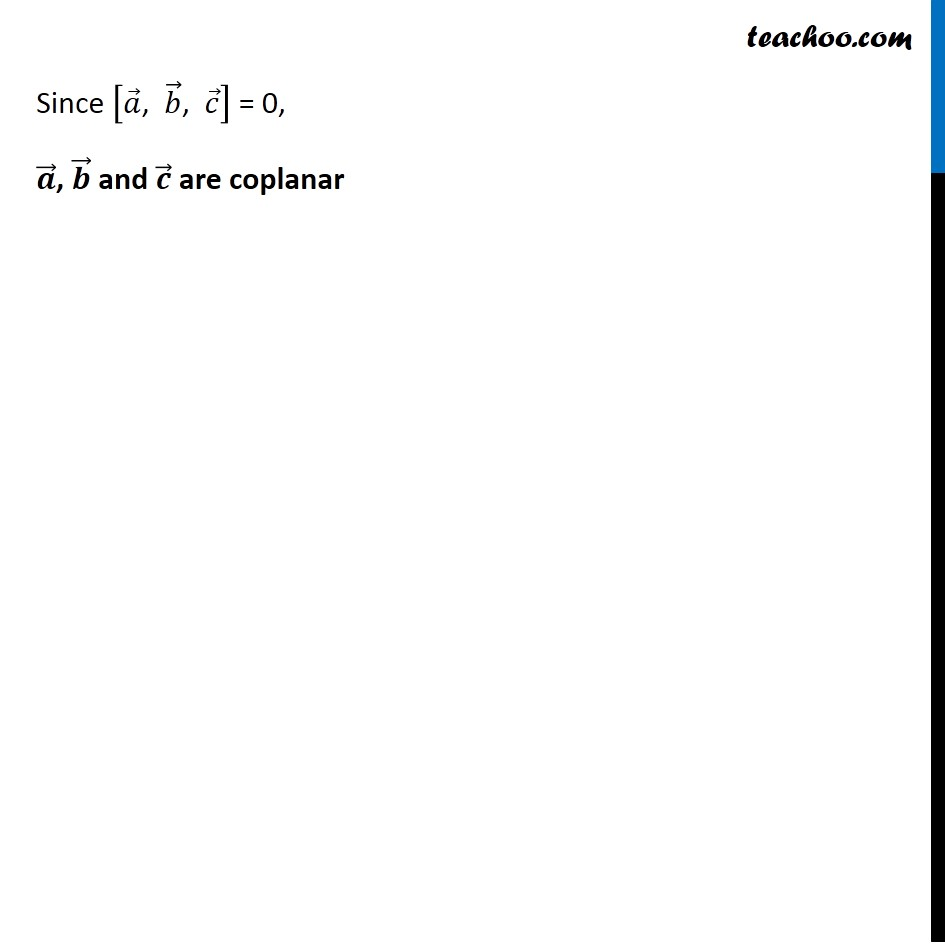Subscribe to our Youtube Channel - https://you.tube/teachoo

1. Chapter 10 Class 12 Vector Algebra
2. Serial order wise
3. Ex 10.5 (Supplementary NCERT)

Transcript

Ex 10.5, 7 (Supplementary NCERT) Show that vectors 𝑎 ⃗, 𝑏 ⃗ and 𝑐 ⃗ are coplanar if 𝑎 ⃗ + 𝑏 ⃗, 𝑏 ⃗ + 𝑐 and 𝑐 ⃗ + 𝑎 ⃗ are coplanarGiven 𝑎 ⃗ + 𝑏 ⃗, 𝑏 ⃗ + 𝑐 and 𝑐 ⃗ + 𝑎 ⃗ are coplanar [■8(𝑎 ⃗" + " 𝑏 ⃗&𝑏 ⃗+𝑐 ⃗&𝑐 ⃗+𝑎 ⃗ )] = 0 We need to prove 𝑎 ⃗, 𝑏 ⃗ and 𝑐 ⃗ are coplanar i.e. [■8(𝑎 ⃗&𝑏 ⃗&𝑐 ⃗ )] = 0 Now, [■8(𝑎 ⃗" + " 𝑏 ⃗&𝑏 ⃗+𝑐 ⃗&𝑐 ⃗+𝑎 ⃗ )] = 0 (𝑎 ⃗" + " 𝑏 ⃗). ["(" 𝑏 ⃗+𝑐 ⃗") " × " (" 𝑐 ⃗+𝑎 ⃗)] = 0 (𝑎 ⃗" + " 𝑏 ⃗). ["(" 𝑏 ⃗ × 𝑐 ⃗") + (" 𝑏 ⃗ × 𝑎 ⃗)+"(" 𝒄 ⃗ × 𝒄 ⃗") + (" 𝑐 ⃗ × 𝑎 ⃗)] = 0 "(" 𝑎 ⃗+𝑏 ⃗")." [(𝑏 ⃗ × 𝑐 ⃗") + (" 𝑏 ⃗ × 𝑎 ⃗ ) "+ 0 + (" 𝑐 ⃗" × " 𝑎 ⃗") " ] = 0 𝑎 ⃗. (𝑏 ⃗ × 𝑐 ⃗) + 𝑏 ⃗.(𝑏 ⃗ × 𝑐 ⃗) + 𝑎 ⃗. (𝑏 ⃗ × 𝑎 ⃗) + 𝑏 ⃗.(𝑏 ⃗ × 𝑎 ⃗) + 𝑎 ⃗.(𝑐 ⃗ × 𝑎 ⃗) + 𝑏 ⃗.(𝑐 ⃗ × 𝑎 ⃗) = 0 "(" 𝑎 ⃗+𝑏 ⃗")." [(𝑏 ⃗×𝑐 ⃗") + (" 𝑏 ⃗×𝑎 ⃗ ) "+ 0 + (" 𝑐 ⃗" × " 𝑎 ⃗") " ] = 0 "(" 𝑎 ⃗+𝑏 ⃗")." [(𝑏 ⃗×𝑐 ⃗") + (" 𝑏 ⃗×𝑎 ⃗ ) "+ (" 𝑐 ⃗" × " 𝑎 ⃗") " ] = 0 𝑎 ⃗. (𝑏 ⃗ × 𝑐 ⃗) + 𝑏 ⃗.(𝑏 ⃗ × 𝑐 ⃗) + 𝑎 ⃗. (𝑏 ⃗ × 𝑎 ⃗) + 𝑏 ⃗.(𝑏 ⃗ × 𝑎 ⃗) + 𝑎 ⃗.(𝑐 ⃗ × 𝑎 ⃗) + 𝑏 ⃗.(𝑐 ⃗ × 𝑎 ⃗) = 0 [𝑎 ⃗", " 𝑏 ⃗", " 𝑐 ⃗ ] + [𝒃 ⃗", " 𝒃 ⃗", " 𝒄 ⃗ ] + [𝒂 ⃗", " 𝒃 ⃗", " 𝒂 ⃗ ] + [𝒃 ⃗", " 𝒃 ⃗", " 𝒂 ⃗ ] + [𝒂 ⃗", " 𝒄 ⃗", " 𝒂 ⃗ ] + [𝑏 ⃗", " 𝑐 ⃗", " 𝑎 ⃗ ] = 0 𝑐 ⃗ × 𝑐 ⃗ = |𝑐 ⃗ ||𝑐 ⃗ | sin 0 𝑛 ̂ = 0 [𝑏 ⃗", " 𝑏 ⃗", " 𝑐 ⃗ ] = [𝑐 ⃗", " 𝑏 ⃗", " 𝑏 ⃗ ] = 𝑐 ⃗. (𝑏 ⃗ × 𝑏 ⃗) As (𝑏 ⃗ × 𝑏 ⃗) = 0 ⃗ = 𝑐 ⃗ . 0 ⃗ = 0 ⃗ Using Prop: [𝑏 ⃗", " 𝑏 ⃗", " 𝑐 ⃗ ] = 0 [𝑎 ⃗", " 𝑏 ⃗", " 𝑎 ⃗ ] = 0 [𝑏 ⃗", " 𝑏 ⃗", " 𝑎 ⃗ ] = 0 [𝑎 ⃗", " 𝑐 ⃗", " 𝑎 ⃗ ] = 0 [𝑎 ⃗", " 𝑏 ⃗", " 𝑐 ⃗ ] + 0 + 0 + 0 + 0 + [𝑏 ⃗", " 𝑐 ⃗", " 𝑎 ⃗ ] = 0 [𝑎 ⃗", " 𝑏 ⃗", " 𝑐 ⃗ ] + [𝒃 ⃗", " 𝒄 ⃗", " 𝒂 ⃗ ] = 0 [𝑎 ⃗", " 𝑏 ⃗", " 𝑐 ⃗ ] + [𝒂 ⃗", " 𝒃 ⃗", " 𝒄 ⃗ ] = 0 2[𝑎 ⃗", " 𝑏 ⃗", " 𝑐 ⃗ ] = 0 [𝑎 ⃗", " 𝑏 ⃗", " 𝑐 ⃗ ] = 0 Since [𝑎 ⃗", " 𝑏 ⃗", " 𝑐 ⃗ ] = 0, 𝒂 ⃗, 𝒃 ⃗ and 𝒄 ⃗ are coplanar

Ex 10.5 (Supplementary NCERT)